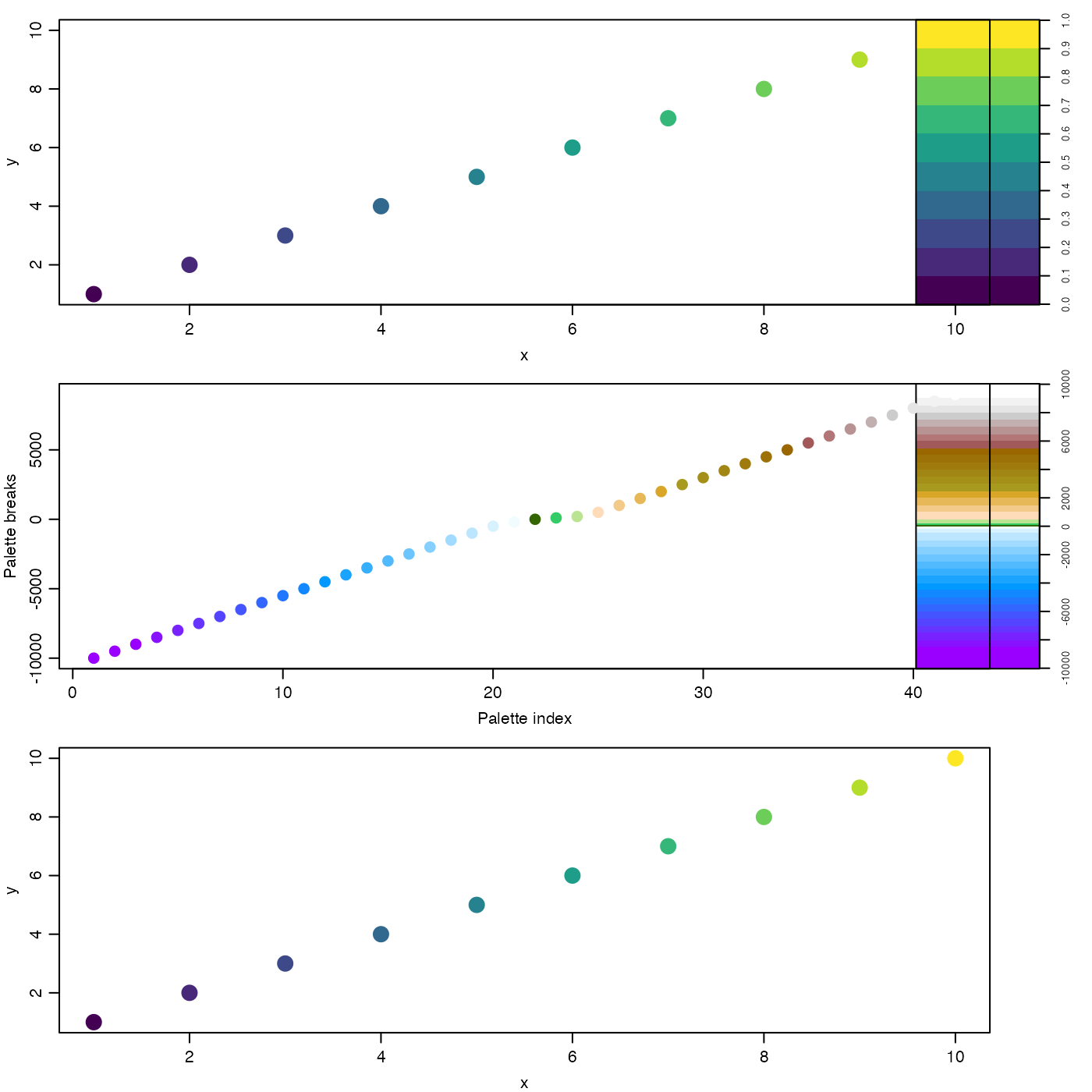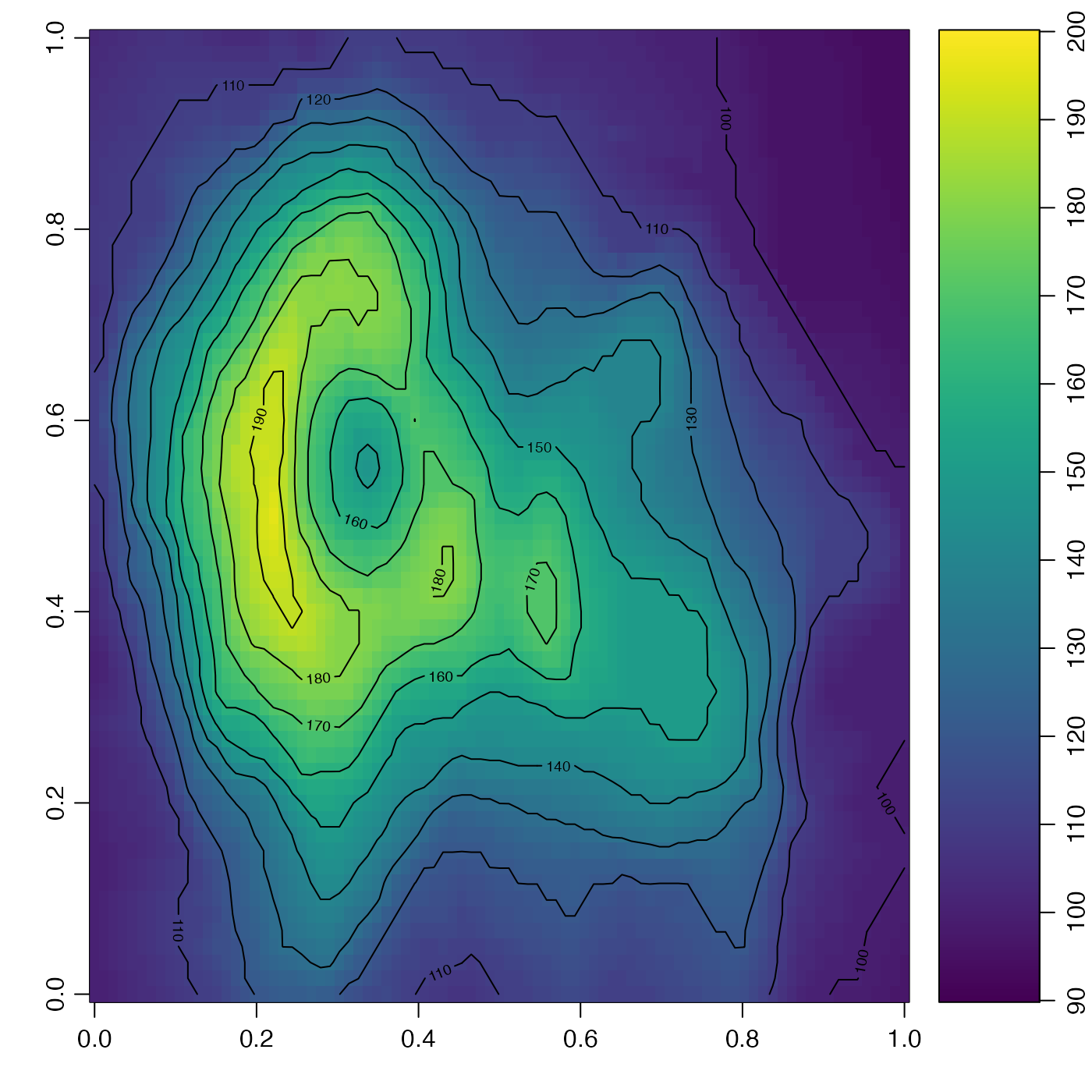In the normal use, drawPalette() draws an image palette near the right-hand side of the plotting device, and then adjusts the global margin settings in such a way as to cause the next plot to appear (with much larger width) to the left of the palette. The function can also be used, if zlim is not provided, to adjust the margin without drawing anything; this is useful in lining up the x axes of a stack of plots, some some of which will have palettes and others not.

drawPalette(
zlim,
zlab = "",
breaks,
col,
colormap,
mai,
cex = par("cex"),
pos = 4,
las = 0,
labels = NULL,
at = NULL,
levels,
drawContours = FALSE,
plot = TRUE,
fullpage = FALSE,
drawTriangles = FALSE,
axisPalette,
tformat,
debug = getOption("oceDebug"),
...
)

## Arguments

zlim two-element vector containing the lower and upper limits of z. This may also be a vector of any length exceeding 1, in which case its range is used. label for the palette scale. the z values for breaks in the color scheme. either a vector of colors corresponding to the breaks, of length 1 less than the number of breaks, or a function specifying colors, e.g. oce.colorsJet() for a rainbow. a color map as created by colormap(). If provided, this takes precedence over breaks and col. margins for palette, as defined in the usual way; see par(). If not given, reasonable values are inferred from the existence of a non-blank zlab. numeric character expansion value for text labels an integer indicating the location of the palette within the plotting area, 1 for near the bottom, 2 for near the left-hand side, 3 for near the top side, and 4 (the default) for near the right-hand side. optional argument, passed to axis(), to control the orientation of numbers along the axis. As explained in the help for par(), the meaning of las is as follows: las=0 (the default) means to put labels parallel to the axis, las=1 means horizontal (regardless of axis orientation), las=2 means perpendicular to the axis, and las=3 means to vertical (regardless of axis orientation). Note that the automatic computation of margin spacing parameter mai assumes that las=0, and so for other cases, the user may need to specify the mai argument directly. optional vector of labels for ticks on palette axis (must correspond with at) optional vector of positions for the labels optional contour levels, in preference to breaks values, to be added to the image if drawContours is TRUE. logical value indicating whether to draw contours on the palette, at the color breaks. logical value indicating whether to plot the palette, the default, or whether to just alter the margins to make space for where the palette would have gone. The latter case may be useful in lining up plots, as in example 1 of “Examples”. logical value indicating whether to draw the palette filling the whole plot width (apart from mai, of course). This can be helpful if the palette panel is to be created with layout(), as illustrated in the “Examples”. logical value indicating whether to draw triangles on the top and bottom of the palette. If a single value is provide, it applies to both ends of the palette. If a pair is provided, the first refers to the lower range of the palette, and the second to the upper range. optional replacement function for axis(), e.g. for exponential notation on large or small values. optional format for axis labels, if the variable is a time type (ignored otherwise). a flag that turns on debugging. Set to 1 to get a moderate amount of debugging information, or to 2 to get more. optional arguments passed to plotting functions.

None.

## Details

The plot positioning is done entirely with margins, not with par(mfrow) or other R schemes for multi-panel plots. This means that the user is free to use those schemes without worrying about nesting or conflicts.

## Use with multi-panel plots

An important consequence of the margin adjustment is that multi-panel plots require that the initial margin be stored prior to the first call to drawPalette(), and reset after each palette-plot pair. This method is illustrated in “Examples”.

This is used by imagep().

## Examples


library(oce)
par(mgp=getOption("oceMgp"))

## 1. A three-panel plot
par(mfrow=c(3, 1), mar=c(3, 3, 1, 1))
omar <- par('mar')                 # save initial margin

## 1a. top panel: simple case
drawPalette(zlim=c(0, 1), col=oce.colorsJet(10))
plot(1:10, 1:10, col=oce.colorsJet(10)[1:10],pch=20,cex=3,xlab='x', ylab='y')
par(mar=omar)                      # reset margin

## 1b. middle panel: colormap
cm <- colormap(name="gmt_globe")
drawPalette(colormap=cm)
icol <- seq_along(cm$col) plot(icol, cm$breaks[icol], pch=20, cex=2, col=cm\$col,
xlab="Palette index", ylab="Palette breaks")
par(mar=omar)                      # reset margin

## 1c. bottom panel: space for palette (to line up graphs)
drawPalette(plot=FALSE)
plot(1:10, 1:10, col=oce.colorsJet(10)[1:10],pch=20,cex=3,xlab='x', ylab='y')par(mar=omar)                      # reset margin

# 2. Use layout to mimic the action of imagep(), with the width
# of the palette region being 14 percent of figure width.
d <- 0.14
layout(matrix(1:2,nrow=1), widths=c(1-d,d))
image(volcano, col=oce.colorsJet(100), zlim=c(90, 200))
drawPalette(c(90, 200), fullpage=TRUE, col=oce.colorsJet)